# 4 5 Graphs of Sine and Cosine Functions

• Slides: 22
Download presentation4. 5 Graphs of Sine and Cosine Functions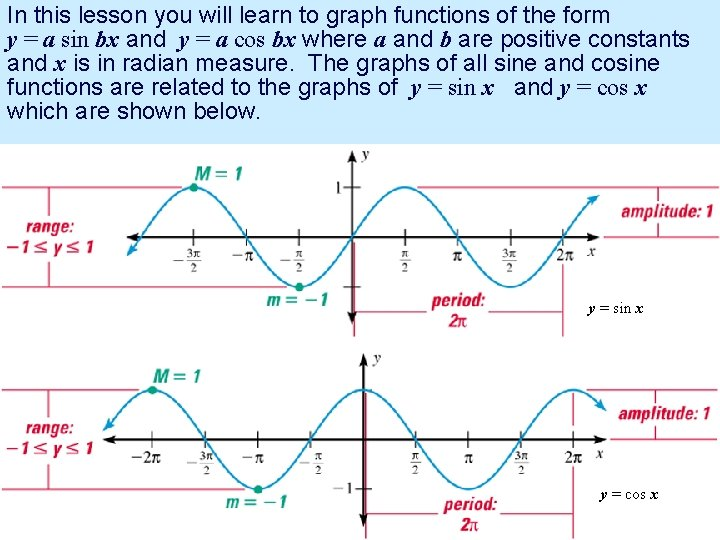In this lesson you will learn to graph functions of the form y = a sin bx and y = a cos bx where a and b are positive constants and x is in radian measure. The graphs of all sine and cosine functions are related to the graphs of y = sin x and y = cos x which are shown below. y = sin x y = cos x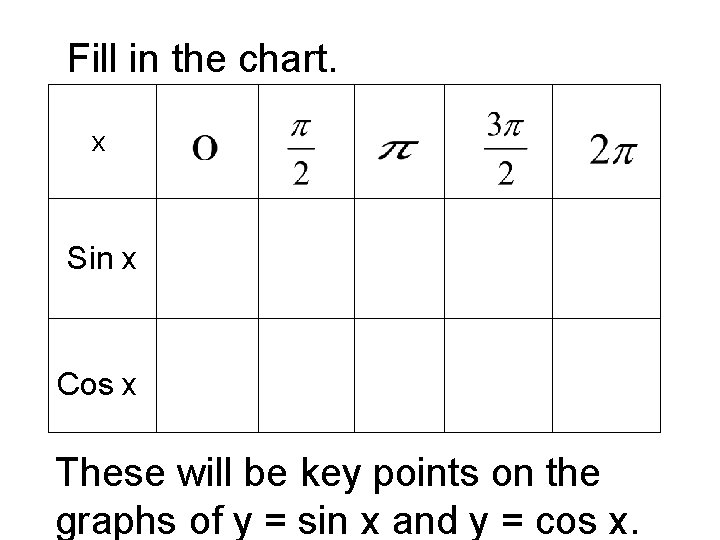Fill in the chart. x Sin x Cos x These will be key points on the graphs of y = sin x and y = cos x.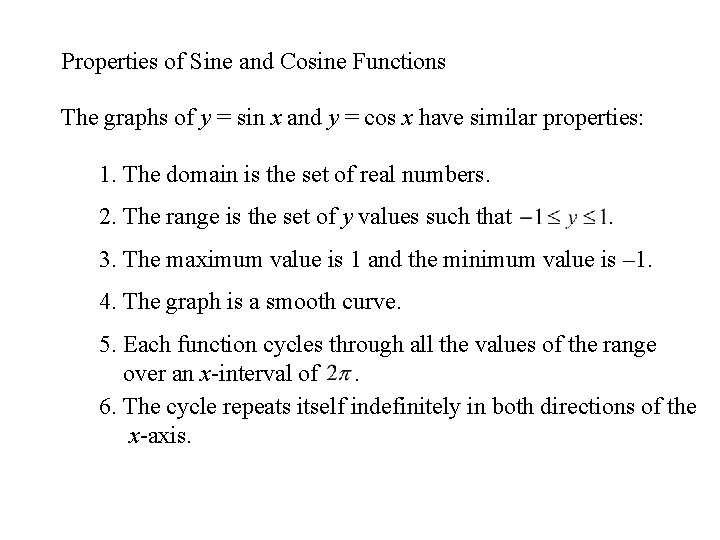Properties of Sine and Cosine Functions The graphs of y = sin x and y = cos x have similar properties: 1. The domain is the set of real numbers. 2. The range is the set of y values such that . 3. The maximum value is 1 and the minimum value is – 1. 4. The graph is a smooth curve. 5. Each function cycles through all the values of the range over an x-interval of. 6. The cycle repeats itself indefinitely in both directions of the x-axis.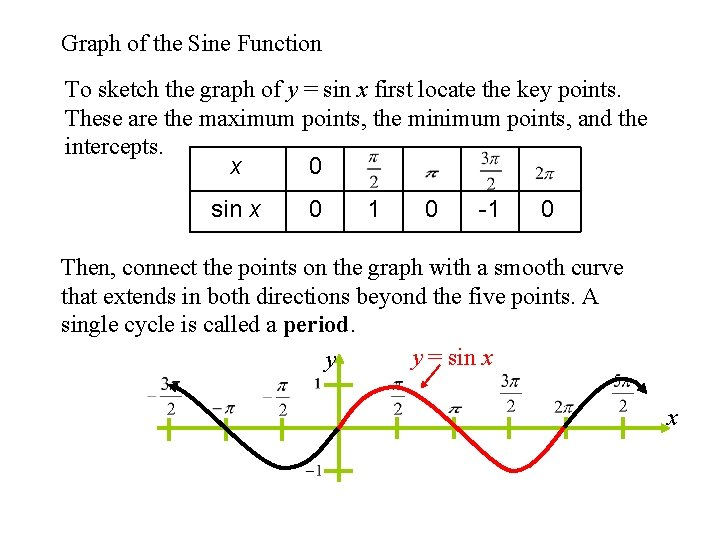Graph of the Sine Function To sketch the graph of y = sin x first locate the key points. These are the maximum points, the minimum points, and the intercepts. x 0 sin x 0 1 0 -1 0 Then, connect the points on the graph with a smooth curve that extends in both directions beyond the five points. A single cycle is called a period. y = sin x y xGraph of the Cosine Function To sketch the graph of y = cos x first locate the key points. These are the maximum points, the minimum points, and the intercepts. x 0 cos x 1 0 -1 0 1 Then, connect the points on the graph with a smooth curve that extends in both directions beyond the five points. A single cycle is called a period. y = cos x y xBefore sketching a graph, you need to know: • Amplitude – Constant that gives vertical stretch or shrink. • Period – • Interval – Divide period by 4 • Critical points – You need 5. (max. , min. , intercepts. )Amplitudes and Periods The graph of y = A sin Bx has amplitude = | A| period = To get your critical points (max, min, and intercepts) just take your period and divide by 4. Example: IntervalThe amplitude of y = a sin x (or y = a cos x) is half the distance between the maximum and minimum values of the function. amplitude = |a| If |a| > 1, the amplitude stretches the graph vertically. If 0 < |a| < 1, the amplitude shrinks the graph vertically. If a < 0, the graph is reflected in the x-axis. y Notice that since all these graphs have B=1, so the period doesn’t change. y = 2 sin x x y= sin x y = – 4 sin x reflection of y = 4 sin x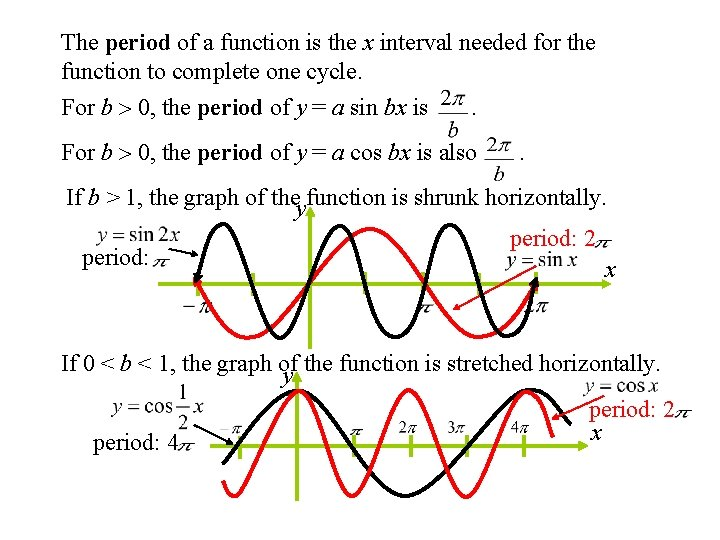The period of a function is the x interval needed for the function to complete one cycle. For b 0, the period of y = a sin bx is . For b 0, the period of y = a cos bx is also . If b > 1, the graph of theyfunction is shrunk horizontally. period: 2 x If 0 < b < 1, the graph of y the function is stretched horizontally. period: 4 period: 2 xExample 1: Sketch the graph of y = 3 cos x on the interval [– , 4 ]. Partition the interval [0, 2 ] into four equal parts. Find the five key points; graph one cycle; then repeat the cycle over the interval. x y = 3 cos x 0 3 max (0, 3) 0 -3 x-int min y ( 0 2 3 x-int max , 3) x ( ( , 0) ( , – 3) , 0)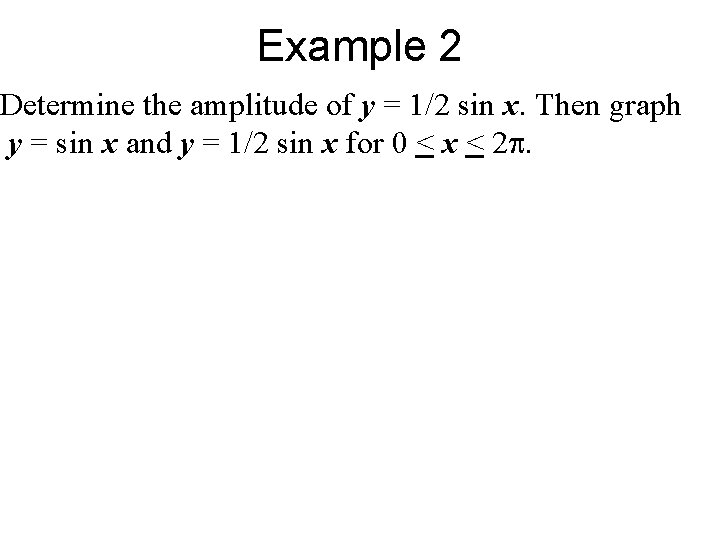Example 2 Determine the amplitude of y = 1/2 sin x. Then graph y = sin x and y = 1/2 sin x for 0 < x < 2.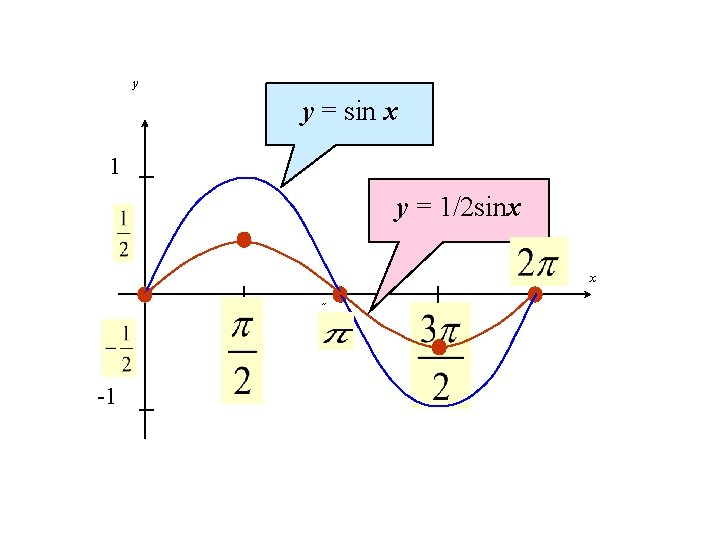y y = sin x 1 y = 1/2 sinx 2˝ x ˝ -1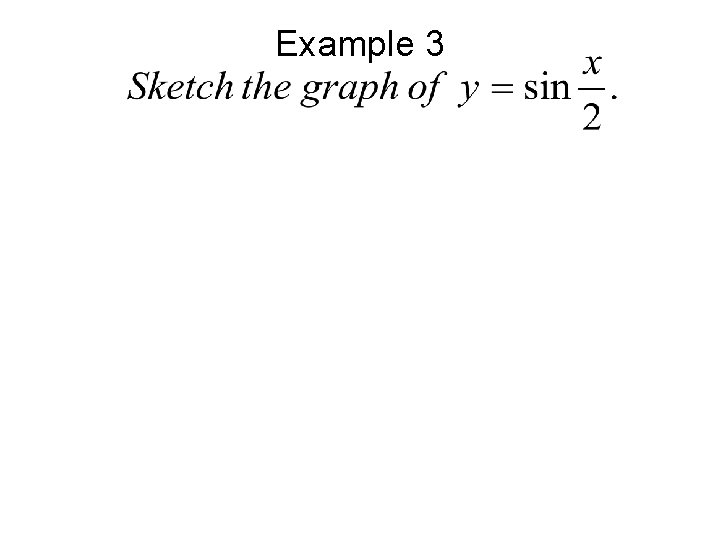Example 3Example 3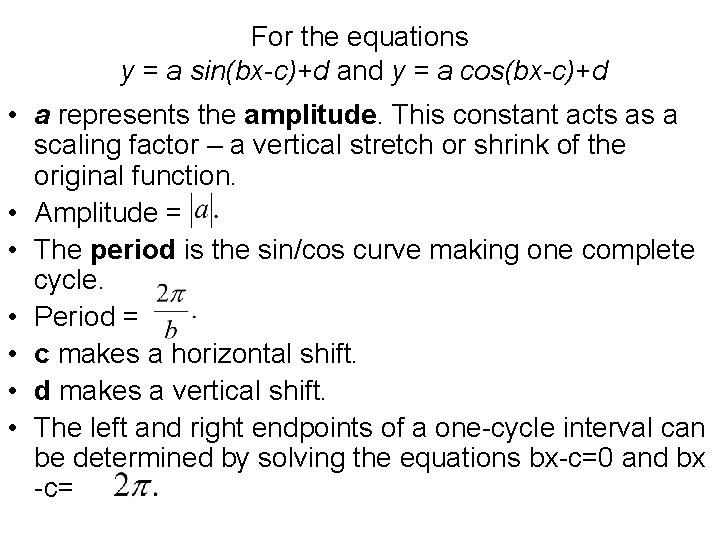For the equations y = a sin(bx-c)+d and y = a cos(bx-c)+d • a represents the amplitude. This constant acts as a scaling factor – a vertical stretch or shrink of the original function. • Amplitude = • The period is the sin/cos curve making one complete cycle. • Period = • c makes a horizontal shift. • d makes a vertical shift. • The left and right endpoints of a one-cycle interval can be determined by solving the equations bx-c=0 and bx -c=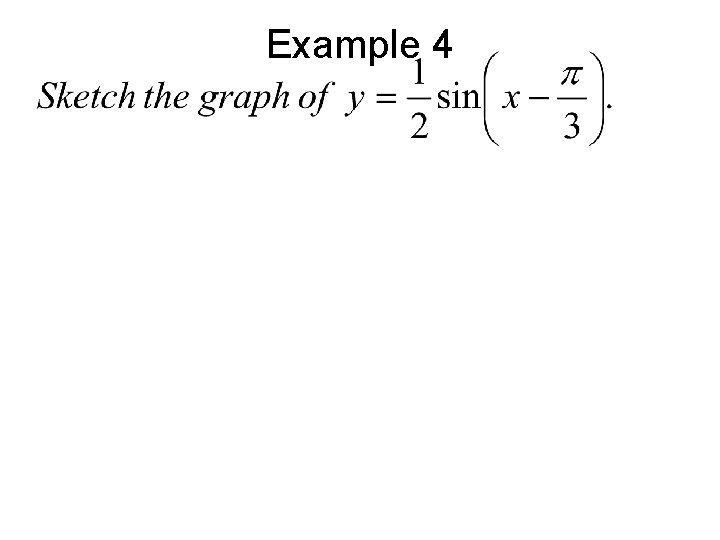Example 4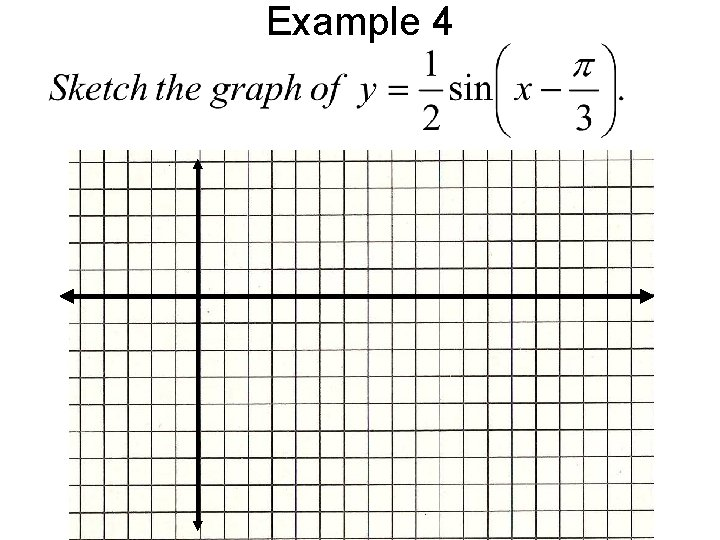Example 4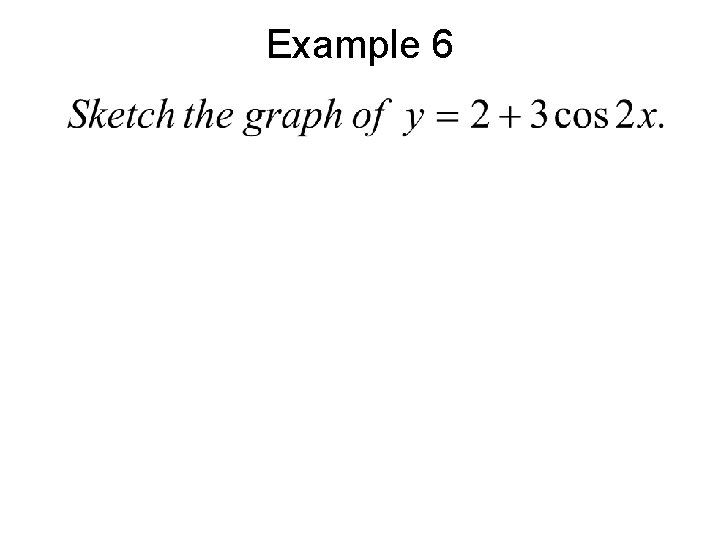Example 6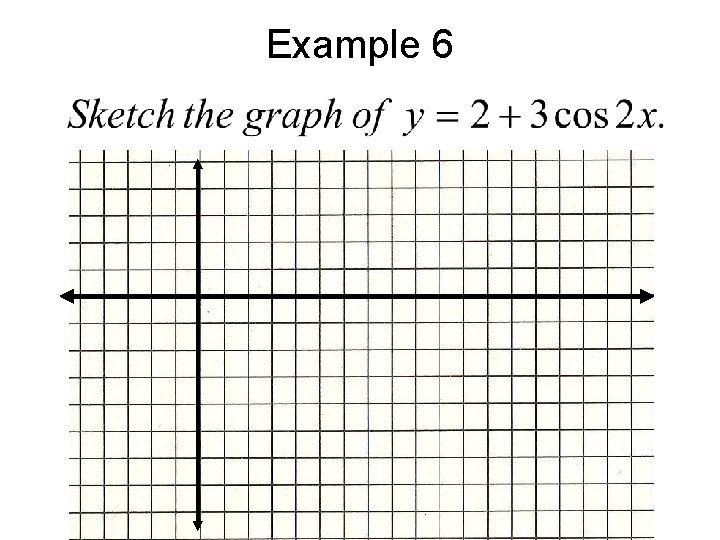Example 6Tides • Throughout the day, the depth of the water at the end of a dock in Bangor, Washington varies with the tides. The tables shows the depths (in feet) at various times during the morning. (a) Use a trig function to model the data. (b) A boat needs at least 10 feet of water to moor at the dock. During what times in the evening can it safely dock? Time Midnight 2 a. m. 4 a. m 6 a. m. 8 a. m. 10 a. m. Noon Depth, y 3. 1 7. 8 11. 3 10. 9 6. 6 1. 7 0. 9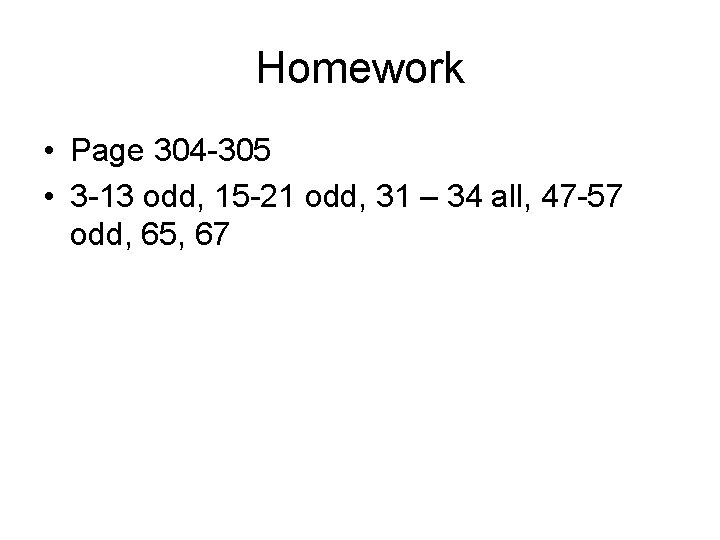Homework • Page 304 -305 • 3 -13 odd, 15 -21 odd, 31 – 34 all, 47 -57 odd, 65, 67